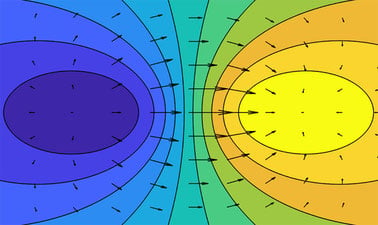# Multivariable Calculus 1: Vectors and Derivatives

We live in a multivariable world. Explore the derivative in higher dimensions and learn how to apply it to solve real world problems. Part 1 of 3.## There is one session available:

After a course session ends, it will be archived.
Estimated 13 weeks
4–6 hours per week
Instructor-paced
Instructor-led on a course schedule

Variables are all around us: temperature, altitude, location, profit, color, and countless others. Multivariable Calculus is the tool of choice to shed light on complex relationships between 2, 3, or hundreds of variables simultaneously.

• How does one control a robot whose motion depends on several variables at once?
• How does an oceanographer understand carbon absorption of the ocean?
• How can one assess if a prediction model matches data optimally?
• How can one design policy to affect the behavior of consumers in order to better protect the planet?

All of these questions involve understanding vectors and derivatives of multivariable functions.

In this course, we begin our exploration of functions of several variables. We will start with learning to visualize multivariable functions, then move to computing and interpreting their derivatives. ​You will discover how to use linear approximations in several variables to simplify complex questions and will start to think about the world through multivariable dependencies.

### At a glance

• Institution: MITx
• Subject: Math
• Level: Intermediate
• Prerequisites:

Single Variable Calculus (basic or undergraduate)

• 18.01.1x
• 18.01.2x (recommended)
• 18.01.3x (recommended)
• Language: English

# What you'll learn

Skip What you'll learn
• How to visualize functions of 2 and 3 variables using level curves and level surfaces.
• How to compute partial derivatives, directional derivatives, and gradients.
• How to optimize multivariable functions subject to constraint equations.
• How to represent the linear approximation of a multivariable function using vectors and matrices.

# Syllabus

Skip Syllabus

Unit 1: Functions of two variables

• Graphing functions and slicing
• Level curves
• Partial derivatives
• Tangent planes
• Linear approximation

Unit 2: Geometry of derivatives

• Vectors
• Dot products
• Vector fields
• Directional derivatives

Unit 3: Optimization

• Critical points
• Second derivative test
• Constrained optimization
• Lagrange multipliers
• Least squares approximation

Unit 4: Matrices

• Systems of equations
• Matrix algebra
• Undetermined and overdetermined systems
• Linear functions as matrix maps

Unit 5: Curves

• Parametric curves in 2 and 3 dimensions
• Chain rule
• Review
• Vectors and derivatives in three dimensions and higher